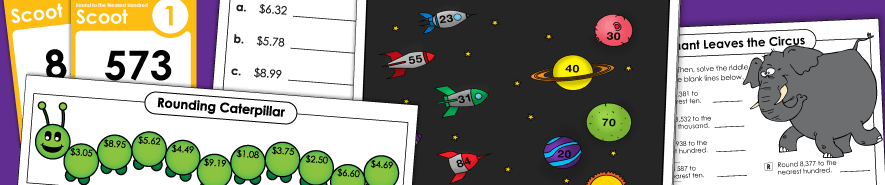# Rounding

The printable worksheets on these pages can help you supplement your math lessons on rounding.  Skills include rounding to the nearest tens, hundreds, thousands, tenths, hundredths, and nearest dollar.## Rounding Whole Numbers

Rounding to Nearest Ten

If you're teaching kids to round 2 and 3-digit numbers to the nearest ten, take a look at this page. It has a Scoot game, lots of worksheets, and rounding charts.
(examples: 75 rounds to 80; 167 rounds to 170)

Rounding to Nearest Hundred

On this worksheets, pupils will round numbers to the nearest hundred. There's also a section for rounding to nearest ten and hundred (mixed).
(examples: 345 rounds to 300; 1,677 rounds to 1,700)

Rounding to Nearest Thousand

This section has a few printables on rounding to the nearest thousand.
(example: 5,765 rounds to 6,000)

## Decimal and Money Rounding

Decimal Rounding

Round decimals to the nearest tenth, hundredth, thousandth, and/or whole number.
(example: 2.67 rounds to 3)

Money Rounding

Round money amounts to the nearest dollar. There's a math riddle activity, and practice worksheets.
(example: \$6.71 rounds to \$7.00)

See also:
Place Value

Super Teacher Worksheets has a HUGE collection of place value worksheets. Use these resources for teaching kids about comparing and ordering numbers, place value blocks, expanded notation, and digit values.

Please Log In to Super Teacher Worksheets
Username:
Password:
Can't remember your password?
My Account
Site Information
Useful LinksNot a Member?
For complete access to thousands of printable lessons click the button or the link below.
© 2021 Super Teacher Worksheets